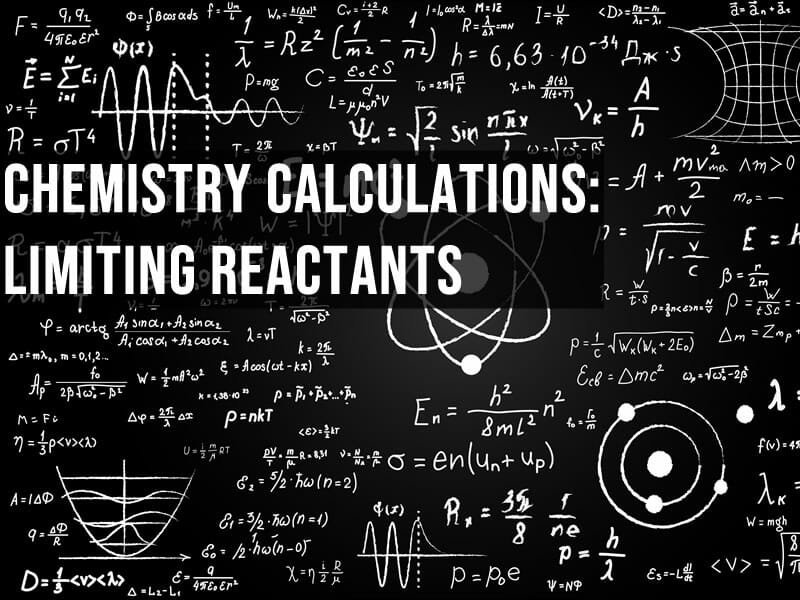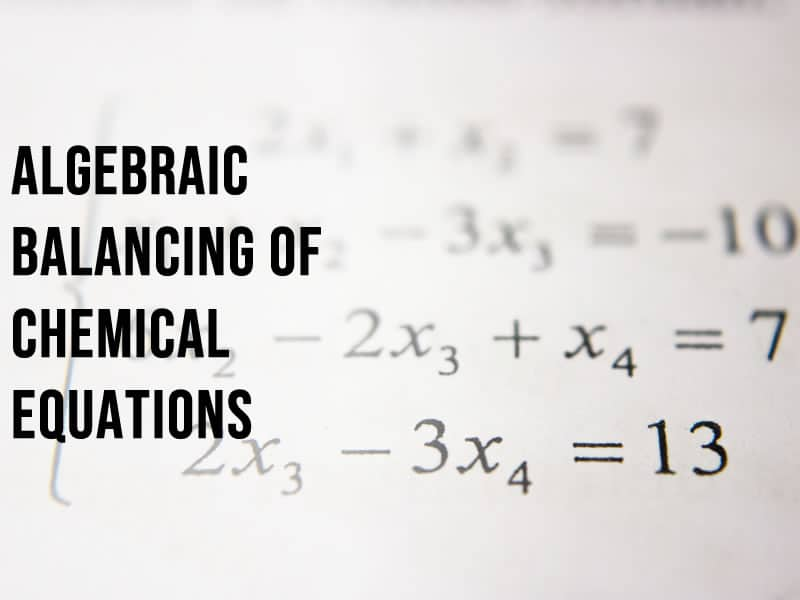# Resources, blog posts and advice

Guides and tutorials about chemistry and teaching2019-04-17T21:30:43+00:00

# All posts

•## Limiting and excess reactants in chemistry

Chemistry calculations: limiting and excess reactants in chemistry The mole has been baffling chemistry students since it was conceived in the nineteenth century so it’s little wonder that the applied concept of limiting reactants confuses students of all levels.  Here are three methods to help you get to grips with limiting reactant calculations. [...]

April 13th, 2020|Categories: Essential Skills, Guides||
•## How to get an A* in A Level Chemistry

How to get an A* in A Level Chemistry Complete understanding of the syllabus, outstanding problem-solving skills, excellent exam technique and a focused plan are the key elements of securing the top grade for A level chemistry. Achieving an A* in A Level chemistry is difficult – only around 1 in 10 [...]

•## Easy way to balance chemical equations for GCSE

Easy way to balance chemical equations for GCSE Balancing chemical equations A chemical equation balancing question is normally worth 1 or 2 marks, which means you have at most 2 minutes to solve it. Everyone is taught the table method when learning to balance chemical equations (where you use a table to track [...]

January 18th, 2019|Categories: Essential Skills, Guides||
•## Is IB chemistry harder than A Level chemistry?

Is IB chemistry harder than A Level chemistry? Chemistry is a popular and essential science for many degrees, so it’s no surprise to find many IB chemistry versus A Level chemistry discussions on the internet debating which programme is the easier route into university. In this blog, I compare the current IB and [...]

January 8th, 2019|Categories: Education, Guides||
•## Balancing chemical equations using algebra

Balancing chemical equations using the algebraic method In the last of my series on balancing chemical equations, we look at the algebraic method, which is useful for balancing the hardest equations Whilst the combination of algebra and balancing chemical equations might sound horrifying, it’s not as bad as it sounds. The algebra [...]

December 3rd, 2018|Categories: Essential Skills, Guides||
•## What are ionic equations and ionic half-equations?

Step-by-step guide to understanding and writing ionic equations Aimed at GCSE, A Level and IB students, this guide will help you master the difficult skills of writing half-equations and ionic half-equations What are ionic equations? Ionic equations are chemical equations that show only ions that participate in a chemical [...]

November 17th, 2018|Categories: Essential Skills, Guides||